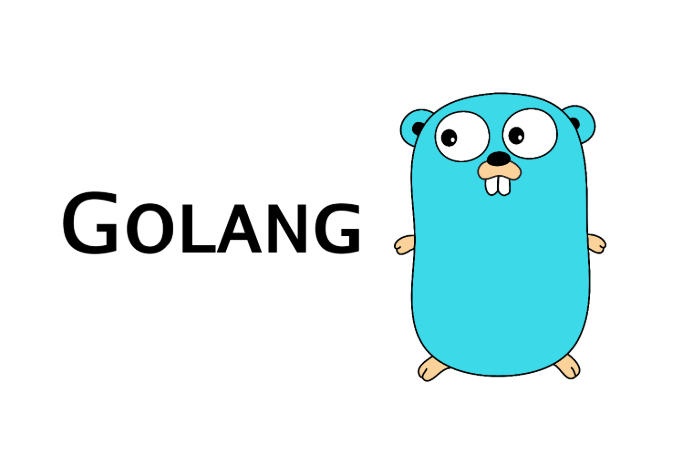Categories

# Go language introductory tutorial (XI)

Original: dry warehouseHello, you small partners Hello everyone, I am a small stack monarch, yesterday spoke about the function of some species go language definitions and custom functions, we then went on to speak of the content continues to share today.

1, no function has a return value of the parameter

Functions that return values, there must be a clear statement of termination, otherwise it will lead to compilation errors. Therefore, the function returns a value requires the use of the keyword return.

He may be one or more return values. Official Suggestion: Best named return value, return value because it is not named, although making the code more concise, but will result in poor readability generated document.

`````` //方式1
func Test01() int {
return 250
}

//方式2, 给返回值命名
func Test02() (a int) {
a = 250
return a
}
//方式3, 给返回值命名
func Test03() (value int) {
value = 250
return
}

func main() {
v1 := Test01() //函数调用
v2 := Test02() //函数调用
v3 := Test03() //函数调用
fmt.Printf("v1 = %d, v2 = %d, v3 = %d\n", v1, v2, v3)
}``````

Had little programming experience may find a partner, such as JavaScript and programming syntax is actually little difference.

For defined functions or calls and other languages ​​are a bit different, if slowly familiar with the syntax, but in fact will go that language is a good place to start, and the high performance type of language.

Why would it say high performance, because the built-go language package offers a lot of performance testing tripartite libraries to help us code tuning work.

So long as we are good at using the tripartite library, good at learning, and will definitely write high-quality, high-availability, high-performance code friends.

Second, no function parameter values ​​of a plurality of return

As the name suggests, is the use of multiple return values ​​Go language processing program to return multiple values, but when the actual development, we usually use two return value is the result of a process that we need to return, and the other is wrong err . To facilitate our exception handling.

``````//方式1
func Test01() (int, string) {
return 520, "it干货栈"
}
//方式2, 给返回值命名
func Test02() (a int, str string) {
a = 520
str = "it干货栈"
return
}

func  main() {
v1, v2 := Test01() //函数调用
_, v3 := Test02()  //函数调用， 第一个返回值丢弃
v4, _ := Test02()  //函数调用， 第二个返回值丢弃
fmt.Printf("v1 = %d, v2 = %s, v3 = %s, v4 = %d\n", v1, v2, v3, v4)
}``````

Third, there are parameters of the function return value

``````//求2个数的和差
func SumAndSubtract(num1 int, num2 int) (sun int, subtract int) {

return num1 +num2 ,num1-num2
}

func main() {
min, max := SumAndSubtract(33, 22)
fmt.Printf("min = %d, max = %d\n", min, max)
//sum = 55, subtract = 11
}``````

Fourth, the function of the recursive function

Recursive function can call itself refers directly or indirectly. Recursive function usually have the same structure: a recursive exit conditions and a body.

The so-called exit conditions is based on the parameters passed to determine whether you need to stop recursion, and recursion body is doing some processing function itself. In a programming language especially for the tree structure we often use is operating on recursive.

We now summed Examples 1 + 2 + 3 + …… 100 through the circulation

``````// 普通实现，利用循环进行累加
func Test01() int {
i := 1
sum := 0
for i = 1; i <= 100; i++ {
sum += i
}

return sum
}

//通过递归实现1+2+3……+100
func Test02(num int) int {
if num == 1 {
return 1
}
return num + Test02(num-1) //函数调用本身
}

//通过递归实现1+2+3……+100
func Test03(num int) int {
if num == 100 {
return 100
}

return num + Test03(num+1) //函数调用本身
}

func main() {
fmt.Println(Test01())    //5050
fmt.Println(Test02(100)) //5050
fmt.Println(Test03(1))   //5050
}``````

We used three ways to implement this example, one is common for the cyclic addition, we have been given the code 100 is a constant dead.

If we make the addition of 99, then you have to change the code, so that practicality is not high.

The other is about the conduct of mass participation, we pass 100 as the final calculation of figures, if we want to make the sum of 50, you only need to change the mass participation, higher availability.

Finally, is the initial mass participation, although can be calculated as less than 100, but if we changed it 101?

He is not going would have been calculated lead to memory overflow it? So when we write code, but also we need to be able to think about, after all, this is a process of accumulation of experience.

Well, today's share on here again, and we shared a moment with no parameters mainly go on the language, there are parameters and recursive functions, the next issue we continue to go about sharing function type language.

I Xiaozhan monarch, if you like my share of trouble you click on a good-looking, forward, or share, of course, can private letter back to me, oh.

Here share includes daily java, go, Python, big data and artificial intelligence-related dry goods, we improve together, progress together. I Xiaozhan monarch, we'll see you tomorrow, thanks to a prayer.